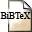# Feasibility and Power Control for Linear Multiuser Receivers in CDMA Networks

## Abstract

This paper is concerned with linear multiuser receivers for code division multiple access (CDMA). Mainly three are of practical relevance: the matched filter (MF), the successive interference cancellation (SIC) and the minimum mean squared error (MMSE) receiver. For the first time, an explicit representation of the signal-to-interference-plus-noise (SINR) ratio for the linear SIC receiver under general random spreading is given. For the MMSE receiver the SINR is obtained by using the asymptotic large system regime as an accurate approximation. Once the SINR for each receiver type is known, the question arises what quality-of-service (QoS) requirements can be supported by an adequate power assignment. A vector of QoS requirements (³i) is called feasible, if there exists some power assignment such that the SINR of each user i does not fall short of threshold ³i. It is shown that for each receiver type there exists a componentwise minimal power assignment such that the SINR of each user equals this threshold. This minimal vector may be determined for the MF and SIC receiver by solving a system of linear equations with coefficients explicitly known. For the MMSE receiver in the large system regime an iterative algorithm is derived, which converges to the optimum power allocation.

## BibTEX Reference Entry```@article{CaMa08,
author = {Daniel Catrein and Rudolf Mathar},
title = "Feasibility and Power Control for Linear Multiuser Receivers in {CDMA} Networks",
pages = "4700-4709",
journal = "{IEEE} Transactions on Wireless Communications",
volume = "7",
number = "11",
month = Nov,
year = 2008,
hsb = RWTH-CONV-223184,
}
```Download paperDownload bibtex-file